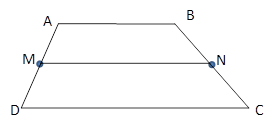Chapter 4.4, Problem 11EElementary Geometry For College St...

7th Edition
Alexander + 2 others
ISBN: 9781337614085

Solutions

Chapter
SectionElementary Geometry For College St...

7th Edition
Alexander + 2 others
ISBN: 9781337614085
Textbook Problem

In Exercises 11 to 16, the drawing shows trapezoid ABCD with A B ¯ | | D C ¯ ; also, M and N are midpoints of A D ¯ and B C ¯ , respectively.Exercises 11-16Given: A B   =   7.3   a n d   D C   =   12.1 Find: M N

To determine

To Find:

MN of trapezoid ABCD.

Explanation

Consider the following trapezoid ABCD.

Given:

AB = 7.3 and DC = 12.1

In the given trapezoid, AB¯||DC¯

Still sussing out bartleby?

Check out a sample textbook solution.

See a sample solution

The Solution to Your Study Problems

Bartleby provides explanations to thousands of textbook problems written by our experts, many with advanced degrees!

Get Started

In Exercises 13-20, sketch a set of coordinate axes and plot each point. 13. (2, 5)

Applied Calculus for the Managerial, Life, and Social Sciences: A Brief Approach

Find the remaining trigonometric ratios. 32. cosx=13,x32

Single Variable Calculus: Early Transcendentals, Volume I

In Exercises 516, evaluate the given quantity. log1,000

Finite Mathematics and Applied Calculus (MindTap Course List)

Find the limit. limh0(h1)3+1h

Single Variable Calculus: Early Transcendentals

An integral for the solid obtained by rotating the region at the right about the y-axis is:

Study Guide for Stewart's Single Variable Calculus: Early Transcendentals, 8th